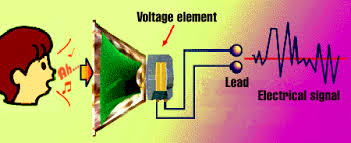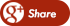﻿ Verilog Examples - Clock Divide by 2Back

## Verilog Examples - Clock Divide by 2Our previous example of cock divide by 2 seemed trivial, so let us extend it to make a divide by 4.So for example if the frequency of the clock input is 50 MHz, the frequency of the output will be 12.5 MHz. In other words the time period of the output clock will be 4 times the time period of the clock input.

The figure shows the example of a clock divider.Problem - Write verilog code that has a clock and a reset as input. It has an output that can be called out_clk. The out_clk is also a clock that has a frequency one forth the frequency of the input clock. It has synchronous reset and if there if the reset is 1, the output clock resets to 0. Write test bench to verify it.

Solution -

This is the main code clock.v

 `module clk_div (clk,reset, clk_out); input clk;input reset;output clk_out; reg [1:0] r_reg;wire [1:0] r_nxt;reg clk_track; always @(posedge clk or posedge reset) beginif (reset)beginr_reg <= 3'b0;clk_track <= 1'b0;end else if (r_nxt == 2'b10)beginr_reg <= 0;clk_track <= ~clk_track;end else r_reg <= r_nxt;end assign r_nxt = r_reg+1; assign clk_out = clk_track;endmodule`